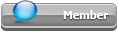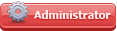## Featured Articles

Check out the latest featured articles.## File Library2# Isenthalpic Throttling With Flashing Liquid

7 replies to this topic
|

### #1lofarcio

lofarcio

Brand New Member

•• Members
•• 4 posts

Posted 06 May 2022 - 03:38 AM

I don't understand why the flow through the throttling valve in cooling cycles is assumed isenthalpic.

The usual justification is that since no moving parts nor heat transfer, then total energy must be constant, and since kinetic energy is similar for similar input-output cross-sectional areas, enthalpy is approximately the same.

But this is only valid for liquids (constant density), and this is not the case in cooling cycles. Since the liquid is close to saturation, it flashes when throttled, and then density falls greatly with the formation of vapor. Then, by continuity, average speed increases also greatly for equal areas.

With sample calculations for R134a between 9 and 2 bars absolute, it can be seen that density falls from ca. 1000 kg/m3 for HP saturated liquid to 36 kg/m3 for 26.7 % LP vapor quality. Then, average speed increases from 1 to 33 m/s, and 32 m/s squared divided by two is 512 J/kg, much more than the latent enthalpy of evaporation.

Under these conditions, how is it that the difference in kinetic energies can be assumed to be negligible?

Sorry if the question has been posted already somewhere or my analysis have great mistakes; I've been unable to find an answer. Any help will be highly appreciated.

### #2latexman

latexman

Gold Member

••• 1,323 posts

Posted 06 May 2022 - 09:00 AM

I don't understand why the flow through the throttling valve in cooling cycles is assumed isenthalpic.

With sample calculations for R134a between 9 and 2 bars absolute, it can be seen that density falls from ca. 1000 kg/m3 for HP saturated liquid to 36 kg/m3 for 26.7 % LP vapor quality. Then, average speed increases from 1 to 33 m/s, and 32 m/s squared divided by two is 512 J/kg, much more than the latent enthalpy of evaporation.

Under these conditions, how is it that the difference in kinetic energies can be assumed to be negligible?

Kinetic energy is not neglected.

Even for the simple case of idea gas flow, we can observe that: Pin > Pout, velin < velout (where P = pressure and vel = velocity). These observations confirm the theory that hin = hout. Remember h = u + Pv (v = specific volume), so if pressure decreases then specific volume must increase if enthalpy is to remain constant (assuming u is constant).

Ditto for more complex two-phase flow.

### #3lofarcio

lofarcio

Brand New Member

•• Members
•• 4 posts

Posted 07 May 2022 - 12:10 PM

Kinetic energy is not neglected.

Even for the simple case of idea gas flow, we can observe that: Pin > Pout, velin < velout (where P = pressure and vel = velocity). These observations confirm the theory that hin = hout. Remember h = u + Pv (v = specific volume), so if pressure decreases then specific volume must increase if enthalpy is to remain constant (assuming u is constant).

Ditto for more complex two-phase flow.

Many thanks for answering, Sir. However, I think your explanation doesn't address my question. You assume enthalpy "is to remain constant", and I am questioning why it must be so from first principles.

If you assume enthalpy is the same input-output, you are assuming kinetic energy difference is negligible. This is so because conservation of total energy says that the difference in enthalpy plus kinetic energy is equal to heat plus (or minus) mechanical work on moving parts. Throttling valves have no moving parts and are usually assumed adiabatic due to their small size. Then, difference of total energy must be zero, and the only way the difference of enthalpy be zero is that also that of kinetic energy be zero.

### #4latexman

latexman

Gold Member

••• 1,323 posts

Posted 07 May 2022 - 06:12 PM

Oh, yes.  I blew it, didn't I.  The problem is not with the definition of enthalpy, it is with the usual simplification of the steady flow energy equation for a throttling process/valve:

H1 + mV12/2 + mgZ1 + Q - W = H2 + mV22/2 + mgZ2

Adiabatic, no work, and negligible kinetic and potential energy yields the usual simplified throttling equation:

H1 = H2

One just has to derive a working equation that is useful for you:

H1 + mV12/2 = H2 + mV22/2

### #5lofarcio

lofarcio

Brand New Member

•• Members
•• 4 posts

Posted 07 May 2022 - 07:38 PM

Oh, yes.  I blew it, didn't I.  The problem is not with the definition of enthalpy, it is with the usual simplification of the steady flow energy equation for a throttling process/valve:

H1 + mV12/2 + mgZ1 + Q - W = H2 + mV22/2 + mgZ2

Adiabatic, no work, and negligible kinetic and potential energy yields the usual simplified throttling equation:

H1 = H2

One just has to derive a working equation that is useful for you:

H1 + mV12/2 = H2 + mV22/2

Thanks again, Sir. I will rephrase my question.

With a liquid, density is constant, so that velocity and kinetic energy are constants too, due to continuity (for a constant cross-section). Then, from the first principle of thermodynamics in an adiabatic flow passage (q = 0, w = 0, so that h + v2/2 = const.), it follows that enthalpy is also a constant.

But in the throttling valve of a refrigeration cycle, the liquid flashes and thus average density decreases greatly, so that continuity tell us that average velocity increases greatly too.

Then my question is:

What is the basis to assume that the variation of average kinetic energy is still negligible, which is the only way to assume that enthalpy continues to be a constant?

### #6breizh

breizh

Gold Member

••• 5,381 posts

Posted 08 May 2022 - 02:11 AM

Hi,

Consider this resource to support your work:

https://www.controlg...ashco_Fluid.pdf

Breizh

### #7latexman

latexman

Gold Member

••• 1,323 posts

Posted 08 May 2022 - 06:24 AM

What is the basis to assume that the variation of average kinetic energy is still negligible, which is the only way to assume that enthalpy continues to be a constant?

H = U + PV

U = internal energy.

PV = the work required to establish the system's physical dimensions, i.e. to make room for it by displacing its surroundings.

In the definition of enthalpy, there is no movement.  It is simple the energy, relative to a reference state, for a substance to exist in it's surroundings.  Therefore, there is no basis that I can see to consider kinetic energy terms in the enthalpy or enthalpy derivation.

There is a basis to consider kinetic energy terms in the steady flow energy equation.

### #8lofarcio

lofarcio

Brand New Member

•• Members
•• 4 posts

Posted 08 May 2022 - 06:26 AM

Hi,

Consider this resource to support your work:

https://www.controlg...ashco_Fluid.pdf

Breizh

Thank you very much, Sir.

### Similar Topics